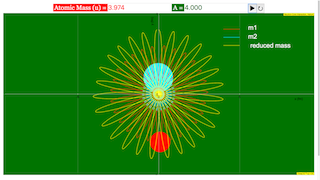Intro Page

nuclear_binary_system_matlab_octave.m - by Javier E. Hasbun and Benjamin E. Hogan (2012)

For a binary system, such as the one shown below, we can use the following equations to determine the motion of m1 and m2:

 r1 = rcm - (m2/M) rr2 = rcm + (m1/M) rrcm = (m1r1+m2r2)/Mμr'' = f r/r μ = m1m2/m1+m2
 Since we know that
 μr'' = f r/r
 we can solve for r'' giving r'' = (f/μ) r/r Seperating this into x- and y-components gives d2x/dt2 = (f/μ)*x/√(x2+y2) d2y/dt2 = (f/μ)*y/√(x2+y2) Next, we need to find the force. Using the WOODS-SAXON spherically symmetric potential which is given by V(r) = -V0*ff(r,R,a) where ff(r,R,a) is a Fermi-function form factor given by ff(r,R,a) = [1+exp(r-R/a)]-1, and the parameters are Vo = 52.06 MeV a = 0.662 fm R = R0*A^(1/3), Ro = 1.26 fm. Since we know that a potential is defined as V(r) = ∫ f(r)∙dr, we can find f(r) by taking the derivative of V(r) w.r.t. r. We get f(r) = -V0*exp(r-R/a)/{a*[1+exp(r-R/a)]}. This is then inserted into the equations of motion giving
 d2x/dt2 = -V0*exp((r-R)/a)*x/{a*μ*[1+exp((r-R)/a)]*√(x2+y2)} d2y/dt2 = -V0*exp((r-R)/a)*y/{a*μ*[1+exp((r-R)/a)]*√(x2+y2)}
 Solving these equations give the x- and y-coordinates of the center of mass which in turn give the x- and y-coodinates of m1 and m2, respectively. To ensure unit compatibility, let x = X*a0 y = Y*a0 a = A*a0 R = R*a0 t = T*tau V0 = V0*Eb m1 = M1*m0 m2 = M2*m0 μ = μ*m0 The equations of motion then become
 d2X/dT2 = -V0*exp((sqrt(X^2+Y^2)-R)/A)*X/{A*μ*[1+exp((sqrt(X^2+Y^2)-R)/A)]^2*√(X2+Y2)} d2Y/dT2 = -V0*exp((sqrt(X^2+Y^2)-R)/A)*Y/{A*μ*[1+exp((sqrt(X^2+Y^2)-R)/A)]^2*√(X2+Y2)} after arbitrarily setting (Eb*tau^2 / m0*a0^2) =1. Solving the above expression for tau, we see that the one second is equivalent to 1.02E-22 seconds of simulation time. To determine the initial conditions, we set t=0, rCM=0, and drCM/dt=vCM=0. This gives r1,0 = -(m2/m1)*r2,0 and v1,0 = -(m2/m1)*v2,0 , or in component form: x1,0 = -(m2/m1)*x2,0
 y1,0 = -(m2/m1)*y2,0
 vx1,0 = -(m2/m1)*vx2,0
 vy1,0 = -(m2/m1)*vy2,0 Setting either component of x=0 makes the y-component a maximum. For y we choose 1. Similarly this would make the y-component of velocity zero, the the x-component would be a maximum. For vx we chose 0.085.

### Translations

Code Language Translator Run### Software Requirements

SoftwareRequirements

 Android iOS Windows MacOS with best with Chrome Chrome Chrome Chrome support full-screen? Yes. Chrome/Opera No. Firefox/ Samsung Internet Not yet Yes Yes cannot work on some mobile browser that don't understand JavaScript such as..... cannot work on Internet Explorer 9 and below

### CreditsJavier E.Hasbun and Benjamin E Hogan; Fremont Teng; Loo Kang Wee

[text]

## Nuclear Binary System Simulator JavaScript Simulation Applet HTML5

### Instructions

#### Atomic Mass and A Field Boxes

Typing in these field boxes will set their atomic mass and A respectively.

#### Toggling Full Screen

Click anywhere in the panel to toggle full screen.

#### Play/Pause and Reset Buttons

Plays/Pauses and resets the simulation respectively.

Research

[text]

[text]

[text]

# Testimonials (0)

There are no testimonials available for viewing. Login to deploy the article and be the first to submit your review!